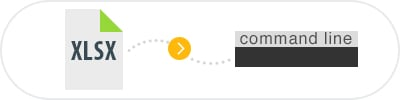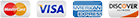Server XLSX Converter

Our flagship Total Excel Converter software is well known as the leading desktop Excel conversion software on the market. But what if you are looking for that kind of power on the server side of the equation?Total Excel Converter X is a full-featured server XLSX converter that you can call remotely or even integrate into your own applications via its support for ActiveX.

Having a server based XLSX converter at your fingertips opens up a world of programmatic power at an incredibly affordable price. Rather than having to reinvent the wheel in your own application, you can simply leverage the decade's worth of testing and user feedback that has already gone into our software.

Here are just a few of the standout features of Total Excel Converter X that should put it at the top of your list of possible purchases:

• ActiveX support allows for integration with any existing web application.
• Converts XLS files quietly and automatically in the background.
• Perfect for tackling large-scale batch conversion tasks.
• Can also be called directly via the command line.

Total Excel Converter X also works with a huge variety of input and output formats so you will be covered across a complete range of common conversion scenarios. The software handles a complete set of XLS, XLSX and OpenOffice formats as input sources along with options such as XML and WK2 files.

If you are looking to convert your files into another structured data format, the software is equipped to handle all common formats. You will be able to convert XLSX to SQL or XLSX to CSV with equal ease.

Sometimes you will be more interested in reading the information rather than manipulating it. If you simply need to extract information for viewing, the option to convert XLSX to PDF will likely be of interest.

When you consider the average hourly cost of custom development work these days, the range of functionality packed into Total Excel Converter X's affordable one-time price is an absolute bargain. You simply will not find a better server-based conversion solution elsewhere on the market.

We encourage you to download a fully functional 30-day free trial to discover its power for yourself prior to purchasing!Examples of Total Excel ConverterX

Convert excel files With TotalExcelConverterX and .NET

string src="C:\\test\\Source.xlsx";
string dest="C:\\test\\Dest.PDF";

ExcelConverterX Cnv = new ExcelConverterX();
Cnv.Convert(src, dest, "-c PDF -log c:\\test\\Excel.log");

MessageBox.Show("Convert complete!");

Convert Excel Files On Web Servers With Total Excel ConverterX

dim C
Set C=CreateObject("ExcelConverter.ExcelConverterX")
C.Convert "c:\test\source.xlsx", "c:\test\dest.pdf", "-cPDF -log c:\test\Excel.log"
Response.Write C.ErrorMessage
set C = nothing
Example2 ASP: directly stream the resulting PDF
dim C
Set C=CreateObject("ExcelConverter.ExcelConverterX")
Response.Clear
Response.BinaryWrite c.ConvertToStream("C:\www\ASP\Source.xlsx", "C:\www\ASP", "-cpdf  -log c:\html.log")
set C = nothing

Convert Excel Spreadsheets by PHP With Total Excel ConverterX

\$src="C:\\test\\test.XLS";
\$dest="C:\\test\\test.CSV";
\$c= new COM("ExcelConverter.ExcelConverterX");
\$c->convert(\$src,\$dest, "-c csv  -log c:\\test\\xls.log");
if (file_exists(\$dest)) echo "OK"; else echo "fail:".\$c->ErrorMessage;

Convert Excel Spreadsheets By Ruby With Total Excel ConverterX

require 'win32ole'
c = WIN32OLE.new('ExcelConverter.ExcelConverterX')

src="C:\\test\\test.xlsx";
dest="C:\\test\\test.pdf";

c.convert(src,dest, "-c PDF -log c:\\test\\Excel.log");

if not File.exist?(dest)
puts c.ErrorMessage
end

Convert Excel Spreadsheets by Python With Total Excel ConverterX

import win32com.client
import os.path

c = win32com.client.Dispatch("ExcelConverter.ExcelConverterX")

src="C:\\test\\test.xlsx";
dest="C:\\test\\test.pdf";

c.convert(src, dest, "-c PDF -log c:\\test\\Excel.log");

if not os.path.exists(file_path):
print(c.ErrorMessage)

Convert Excel files With Pascal and Total Excel ConverterX

uses Dialogs, Vcl.OleAuto;

var
c: OleVariant;
begin
c:=CreateOleObject('ExcelConverter.ExcelConverterX');
C.Convert('c:\test\source.xlsx', 'c:\test\dest.pdf', '-cPDF -log c:\test\Excel.log');
IF c.ErrorMessage<> Then
ShowMessage(c.ErrorMessage);
end;

Convert Excel and ODS Files On Web Servers With Total Excel ConverterX

var c = new ActiveXObject("ExcelConverter.ExcelConverterX");
c.Convert("C:\\test\\source.xlsx", "C:\\test\\dest.pdf", "-c PDF");
if (c.ErrorMessage!="")

Convert Excel Spreadsheets by Perl With Total Excel ConverterX

use Win32::OLE;

my \$src="C:\\test\\test.XLS";
my \$dest="C:\\test\\test.CSV";

my \$c = CreateObject Win32::OLE 'ExcelConverter.ExcelConverterX';
\$c->convert(\$src,\$dest, "-c csv  -log c:\\test\\xls.log");
print \$c->ErrorMessage if -e \$dest;

Latest News

• New Total PDF Printer has been released. Now you can print a folder of PDF files and then move the files to a different folder (-msuccess).
• We have fixed an issue in Coolutils Converter where some messages in HTML were not showing correctly.
• New Total HTML Converter has got an update with a few important bug fixes (large radius, paged layout).
• New Total Mail Converter Pro offers enhanced option to fit extra wide tables from emails to PDF files.
• New PDF Combine 7.0 is live. New options for creating combined PDF scans, e-books and songbooks.
• New Total Excel Converter 6.0 is live. Convert XLS with with charts.